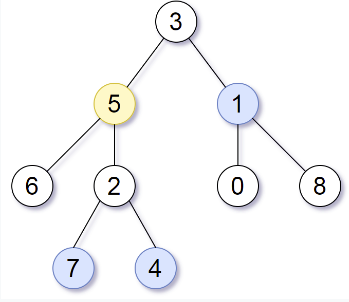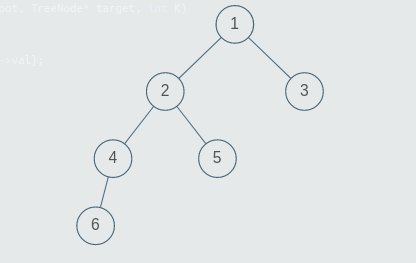New update is available. Click here to update.Close
Topic list
Print Nodes at Distance K From a Given Node
HARD
20 mins12 upvotes
Trees
Topics (Covered in this problem)
Problem solved
Skill meterTrees
-
-
Other topics
Problem solved
Skill meterStrings
-
-Matrices (2D Arrays)
-
--
-Sorting
-
-Binary Search
-
-Stacks & Queues
-
-Graph
-
-Dynamic Programming
-
-Greedy
-
-Tries
-
-Arrays
-
-SQL
-
-Binary Search Trees
-
-Heap
-
-Bit Manipulation
-
-
Solve problems & track your progress
Checkout your overall progress in every topic here
BecomeSensei
in DSA topics
Open the topic and solve more problems associated with it to improve your skills
Check out the skill meter for every topic
See how many problems you are left with to solve for cracking any stage. Score more than zero to get your progress counted.

# Print Nodes at Distance K From a Given Node

Hard0/120
Avg time to solve 20 mins
Success Rate 80 %Share12 upvotes

## Problem Statement

#### Note:

``````1. A binary tree is a tree in which each node has at most two children.
2. The given tree will be non-empty.
3. The given tree can have multiple nodes with the same value.
4. If there are no nodes in the tree which are at distance = K from the given node, return an empty list.
5. You can return the list of values of valid nodes in any order. For example if the valid nodes have values 1,2,3, then you can return {1,2,3} or {3,1,2} etc.
``````
Detailed explanation ( Input/output format, Notes, Constraints, Images )##### Sample Input 1 :
``````1
3 5 1 6 2 0 8 -1 -1 7 4 -1 -1 -1 -1 -1 -1 -1 -1
5
2
``````
##### Sample Output 1 :
``````7 4 1
``````
##### Explanation For The Sample Output 1:``````Target Node is 5. Nodes at distance 1 from 5 are {6, 2, 3} and nodes at distance 2 are {7, 4, 1}.
``````
##### Sample Input 2:
``````1
1 2 3 4 5 -1 -1 6 -1 -1 -1 -1 -1
5
3
``````
##### Sample Output 2:
``````6 3
``````
##### Explanation For The Sample Output 2:``````The tree will be generated as above. The target node is 5 and K = 3. The nodes at distance 1 from node 5 are {2}, nodes at distance 2 from node 5 are {1, 4} and nodes at distance 3 from node 5 are {6, 3}.
``````AutoConsole